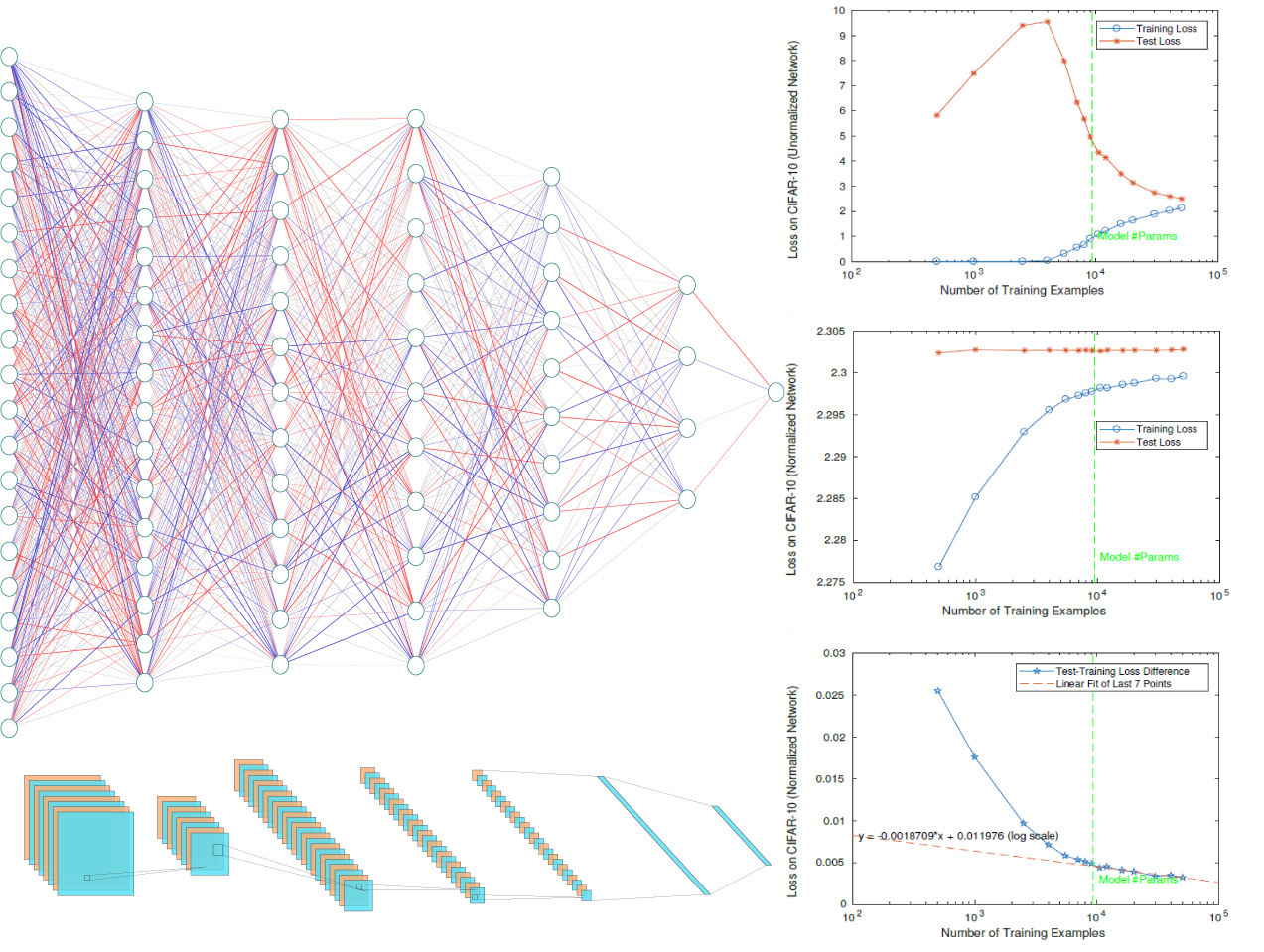# Deep networks are not too complex

Moving away from alchemy into the age of science for deep learningImagine you’re back in elementary school and just took your first statistics course on fitting models to data. One thing you’re sure about is that a good model surely should have less parameters than data (think of fitting ten data points with a line, i.e. two parameters), otherwise you’ll ruin the predictivity of your model by overfitting. But then deep learning shows up, where the best practice seems to be perfectly fitting your training data with many more parameters – so many in fact that you can fit random noise with your model (think of fitting ten data points with a polynomial of a thousand coefficients). How can this be? Do we need to completely rethink the basics of statistical learning theory?Training and test error in the CIFAR 10 dataset as a function of number of neurons in a 5-layer convolutional network. The expected classification error on unseen data does not get worse when we increase the number of parameters beyond the size of the training set.

This is a question we set out to answer in our new Nature Communications paper. Our suspicions were that no magic would be necessary to understand deep nonlinear neural networks, and so we turned our attention first to understanding how exactly the classic case of linear problems works. It has been known for some time that in linear systems that have more unknowns than equations there are infinitely many solutions. However, if we try solving this system by the technique of gradient descent (which is what we also use to train deep nets), while imposing a constraint that the “size”, or norm, of our parameters doesn’t grow too big, we find a solution known as the Moore-Penrose pseudo-inverse. This turns out in practice to work remarkably well - in fact it’s the best known solution in linear regression that does well on unseen data. The problem we faced however is that deep networks work well even without imposing any such constraints!

A small, entirely skippable, technical aside for the curious reader: whether our machine learning model will overfit to the training data actually depends on how “complex” the class of models is. This complexity can be measured in multiple ways, many of them less naive than just straightforward parameter counting, with some of the more often used being Vapnik–Chervonenkis (VC) dimension, covering numbers, or Rademacher complexity. What is important to understand about these measures of complexity is that they do depend on the size of the parameters of the model, and hence a constraint on this norm should imply less overfitting. The question then is “where is this hidden complexity control?”

We found our inspiration in the work of the group led by Nati Srebro, in which they managed to show that in the case of linear classification something nearly magical happens. In these problems one optimizes an exponential-type loss (or cost) function. Two interesting things happen when this optimization is done with the gradient descent technique: first, the size of the parameters in the model keeps increasing indefinitely the longer you run it (eventually becoming infinite); second, the direction in which gradient descent leads is that of the maximum classification margin solution, which is the same as that of the constrained regression problem. This means you can find the equivalent of the pseudo-inverse without any explicit constraint in place!

What we managed to show in our paper is that a similar result holds for deep networks. We observed that the model parameters themselves do not matter for classification, but only their normalized (i.e. unit size) versions matter and that we get this constraint from their simple implicit definition. What was not obvious was that the dynamics of learning in these normalized parameters induced by standard gradient descent would converge anywhere useful. We then felt pretty happy to find that it does, and does so to solutions that locally maximize the classification margin, just like in the linear case of the pseudo-inverse (which does not care about the number of parameters).

An exciting (and surprising to us) result we stumbled upon while doing all of this was that the learning dynamics of standard gradient descent and that of a commonly used technique of “weight normalization” were only different by a square of the size of the parameters. A quick calculation showed us that this meant the latter converges much faster, but more importantly put us in an experimental mindset: maybe we can do even better, since if the size of the parameters only matters to the speed of convergence, we can design new algorithms that manually scale this size and lead to greatly reduced training times. Stay tuned for the sequel!